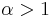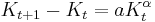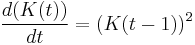# Difference between revisions of "Stylized verbal model of long-run economic growth"

This page describes typical aspects of long-run economic growth models. The simplest model along the lines described here is the Harrod-Domar model. More sophisticated models include the Solow-Swan model, Mankiw-Romer-Weil model, Ramsey-Kass-Coopmans model, and AK model.

## Basic aspects of the model

• Inputs (such as labor, capital, and technology) that could vary from year to year, determine outputs at a given point of time (or in a given year), via a fixed functional relationship (called a production function). The production function itself is fixed.
• The inputs themselves can change from year to year. Part of this change is through feedback from the output: some of the output gets allocated to investment such as investment in physical capital, investment in human capital (either through population growth or through improving the skill level of the existing population), or investment in technological improvement. The extent to which this happens depends on the economy's savings rate. However, there could also be changes to inputs arising from other factors (such as serendipitious discovery of new technology, or discovery of new natural resources, or other "random chance" factors).

## Continuous and discrete models

### Basic distinction

Discrete models use fixed time intervals, such as years. In a given year, the output is determined from the inputs via a production function. The part of the output that gets saved then determines changes to the inputs for next year, which in turn determine the output for that year, and so on. To "solve" this type of model given some initial conditions, we simply compute the inputs and outputs for successive years one at a time.

In continuous models, the output per unit time is computed using the production function of the input per unit time. Further, the rate of change of inputs with respect to time (formally given as the derivative of the inputs with respect to time) is expressed in terms of the current values of inputs and outputs. We thus obtain a differential equation. We can then solve this differential equation using mathematical techniques and thereby get a picture of how the economy grows over time.

### Caveat about converting a discrete model to the analogous continuous model

Naively, one might expect that the discrete model:

(Change in input from one year to the next) = (Some expression$F$ involving inputs and outputs)

and the continuous model:

(Rate of change in input from one year to the next) = (The same expression$F$ involving inputs and outputs)

lead to the same qualitative predictions. And this is true when$F$ is relatively "small" but fails when$F$ grows fast. In the latter case, continuous models predict much faster growth rate than discrete models. The reason, roughly, is that continuous models allow for very rapid feedback from inputs to outputs, that escalates rather quickly, whereas discrete models enforce a year's time lag regardless of how quickly the inputs and outputs are growing. In particular, consider a model of the form:$\frac{dK}{dt} = aK^\alpha$

where$\alpha > 1$. This has an asymptote at finite time. On the other hand, the discrete version:$K_{t+1} - K_t = aK_t^\alpha$

grows superexponentially, but does not reach infinity in finite time.

### Delay differential equations: combining the lagged nature of discrete models with the infinite divisibility of continuous models

A delay differential equation is a variant of the concept of differential equation where one is allowed to use the value of a variable a fixed amount of time in the past. In the context of the economic growth model, a delay differential equation might be something like:$\frac{d(K(t))}{dt} = (K(t - 1))^2$

This says that the rate of growth of the capital stock is proportional to the square of the capital stock one time unit ago (i.e., one year ago, if time is measured in years) ago. Delay differential equations therefore capture the lagged nature of discrete models while still allowing for continuous change.